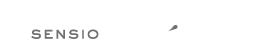# Rule `short_scalar_cast`¶

Cast `(boolean)` and `(integer)` should be written as `(bool)` and `(int)`, `(double)` and `(real)` as `(float)`, `(binary)` as `(string)`.

## Examples¶

### Example #1¶

```--- Original
+++ New
<?php
-\$a = (boolean) \$b;
-\$a = (integer) \$b;
-\$a = (double) \$b;
-\$a = (real) \$b;
+\$a = (bool) \$b;
+\$a = (int) \$b;
+\$a = (float) \$b;
+\$a = (float) \$b;

-\$a = (binary) \$b;
+\$a = (string) \$b;
```

### Example #2¶

```--- Original
+++ New
<?php
-\$a = (boolean) \$b;
-\$a = (integer) \$b;
-\$a = (double) \$b;
+\$a = (bool) \$b;
+\$a = (int) \$b;
+\$a = (float) \$b;

-\$a = (binary) \$b;
+\$a = (string) \$b;
```

## Rule sets¶

The rule is part of the following rule sets:

@PER

Using the @PER rule set will enable the `short_scalar_cast` rule.

@PHP74Migration

Using the @PHP74Migration rule set will enable the `short_scalar_cast` rule.

@PHP80Migration

Using the @PHP80Migration rule set will enable the `short_scalar_cast` rule.

@PHP81Migration

Using the @PHP81Migration rule set will enable the `short_scalar_cast` rule.

@PHP82Migration

Using the @PHP82Migration rule set will enable the `short_scalar_cast` rule.

@PSR12

Using the @PSR12 rule set will enable the `short_scalar_cast` rule.

@PhpCsFixer

Using the @PhpCsFixer rule set will enable the `short_scalar_cast` rule.

@Symfony

Using the @Symfony rule set will enable the `short_scalar_cast` rule.

The logo is © 2010-2022 Sensio Labs